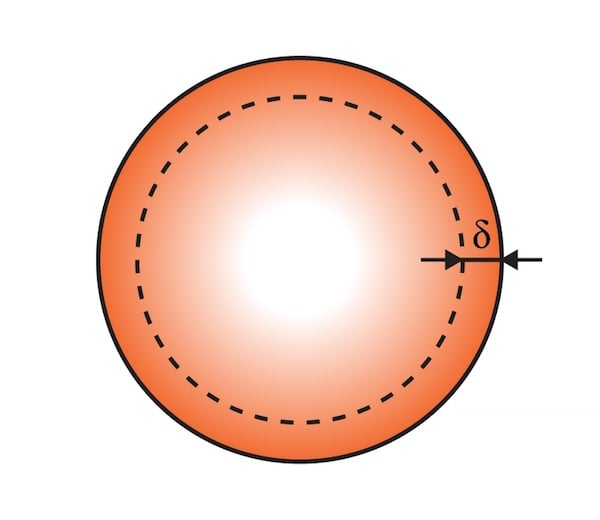# Skin Depth Calculator

## A tool designed to calculate skin depth based on the conductive material being used and the frequency of the signal.

(μΩ cm)

(μm)

### What is the Skin Effect?

The skin effect is a phenomenon whereby alternating electric current does not flow uniformly with respect to the cross-section of a conductive element, such as a wire. The current density is highest near the surface of the conductor and decreases exponentially as distance from the surface increases.

"Skin depth" refers to the point at which the current density reaches approximately 37% of its value at the surface of the conductor. Calculating skin depth requires the frequency of the AC signal and the resistivity and relative permeability of the conductive material. To use this calculator, just select the material type and enter the signal frequency. The resistivity and relative permeability of the chosen material will be automatically given.### Equations for Calculating Skin Depth

$$\delta = \sqrt{\frac{\rho}{\pi f_{o} \mu_{r} \mu_{o}}}$$

Where:

$$\delta$$ = skin depth

$$\rho$$ = resistivity

$$f_{o}$$ = signal frequency

$$\mu_{r}$$ = relative permeability

$$\mu_{o}$$ = permeability of free space = 4π x 10-7

### Applications of Skin Depth

Skin depth is a convenient way to identify the region of the conductor in which the majority of current will flow. It is unnecessary (or in some cases wasteful) to use a wire with a radius that is significantly larger than the skin depth, because most of the current flows in the skin-depth region regardless of the size of the conductor.

The concept of skin depth might be better appreciated with the help of a real-world example. Consider RF signals for WiFi or Bluetooth, which operate at 2.4 GHz. Using the calculator, we see that the skin depth with a copper conductor is 1.331 micrometers. This means that even with a very thin (e.g., 30 AWG) wire, only a tiny fraction of the wire is carrying a significant amount of current.